Courses

Introduction to Ionic Equilibrium Notes | Study Physical Chemistry - Chemistry

Chemistry: Introduction to Ionic Equilibrium Notes | Study Physical Chemistry - Chemistry

The document Introduction to Ionic Equilibrium Notes | Study Physical Chemistry - Chemistry is a part of the Chemistry Course Physical Chemistry.
All you need of Chemistry at this link: Chemistry

Ionic Equilibrium

Electrolytes in solution conducts electricity Because of two reasons:

• Free ions

• Free electrons

Conductors:

Substances which show conductivity or flow of current are called conductors.

Metallic conductor:

These substances show conductivity due to motion of free electrons.

Ex.: Graphite

Ionic Conductor:

These substances which show conductivity due to movement of free ions.

Ionic conductors are further classified into two types:

Strong electrolytes

Those ionic conductors which are completely ionized is aqueous solution are called strong electrolyte.

Ex: Na+Cl

Strong Acid: H2SO4, HCl, HNO3                  Strong Base: KOH, NaOH

All Salts:NaCl, KCl                                       α = 1

Weak Electrolytes:

Those electrolytes which doesn’t completely ionized in aqueous solutions rather only partially ionized are called weak electrolytes.

α < 1   (generally)

1. Weak acid – HCN, CH3 COOH

2. Weak Base – NH4OH, Zn(OH)2, Ca(OH)2

Acids Bases and Salts

Arrhenius Concept:

Arrhenius Acid: according to Arrhenius concept, an acid is a substance that dissociates to given hydrogen ions when dissolved in water

HCl (g) + Water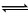H+ (aq) + Cl (aq)

The symbol aq indicates that the ions are hydrated i.e., associated with one or more water Molecules.

Arrhenius Base: According to Arrhenius concept, a base is a substance that dissociated into Hydroxy / ions when dissolved in water.

Ca(OH)2 + WaterCa2+(aq) + 2OH (aq)

The greater the number of H+ or OH ions given by an acid or a base in water, the greater is the strength of the acid or the base.

2. Proton Transfer Theory:

Concept of Lowry and Bronsted According to their concept – “An acid is defined as substance which has a tendency to donate a proton to any other substance and a base is a substance which has a tendency to accept a proton from any other substance”

In other words, Acid is a proton donor and a base is proton acceptor.

Conjugate Acids and Bases

HX      +          BHB+     +          X

Acid                base                 conjugate         conjugate
acid                   base

The limitation of the Bronsted Lowry Theory is that the extent to which a dissolved substance can act as an acid or a base depends largely on solvent.

3. Lewis Concept:

An acid is a molecule / ion which can accept an electron pain with the formative of a coordinated bond.

Ex: BF3, AlCl3

A base is any Molecule which has a lone pair of electrons which can be donated.

Ex: NH3, PH3, OH

Few acid: base reactions are:

(1)        BF3      +          NH3     →        F3B←NH3

Acid                Base

(2)        Cu2+     +          4CN    →         [Cu(CN)4]2–

Acid                Base

Degree of Dissociation (α)

As the Name suggest, α tells us the extent of dissociation. When an electrolyte is dissolved in a solvent H2O, it spontaneously dissociates into ions.

The degree of dissociation (α) of an electrolyte is the fraction of one mole of the electrolyte that has dissociated under the given conditions.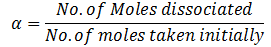Dissociation of weak acid:

HA1H + A

t = 0                             C                     –         –

t = teq                          C – Cα                        Cα    Cα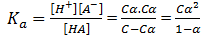2 + αka – ka = 0

Suppose, α <<< 1

Hence 1 – α ≈ 1

Ka = Cα2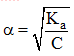This Expression is after neglection when α < 0.05. Otherwise, solve quadratic equation to find the value of α.

Factors affecting the value of degree of dissociation

1. Ostwald’s Dilution Law: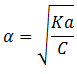As dilution increases, concentration decreases which ultimately leads to increase in degree of dissociation.

1. Temperature

We know, dissociation is endothermic Reaction. ΔH = +ve. Hence, when temperature increases then Keq increases.

Ka =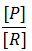As Keq increases, conc.Of product increases. Ultimately degree of dissociation (Δα) increases:

Points to Consider:

Seen Ions: When weak acid HA dissociates

HAH++ A

C–Cα              Cα   Cα

[HA] = C – Cα

[H+] = Cα

After Neglection, α =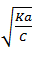Hence [H+] =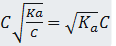For pH of solution, you need [H+]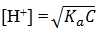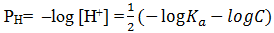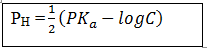when α <<< 1

Hidden Ions: Dissociation of H2O also takes place

H2OH+ + OH

Molarity =       55.55 M

[H+] [OH] = 10–14

If [H+] from acid is greater than 10–6.Then can we neglect dissociation of water.

But otherwise, we have to consider dissociation of water also.

Note: Always keep in mind, while dissociation of any electrolyte. Water is always present. It’s just about neglect ion depending upon the criteria of Conc. of Hydrogen ions when we compare acid and water.

Example: For a weak acid HA, the acid dissociation constant is 10–5. If the concentration of weak acid is 0.1 M.

1. Find the degree of dissociation

2. Concentration of H+

3. Concentration of weak acid

4. Concentration of hydroxyl ion

5. Find the PH.

Solution          HAH+ A

At t = 0           C                   –       –

C – Cα                        Cα   Cα

Kα =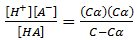Kα = Cα2         [1 – α ≈ 1]

α =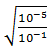= 10-2

α, we have calculated is less than .05. Hence our neglection was right.

2)   [H+] = Ca = 10–1 x 10–2 = 10–3

3) Conc. of weak acid

[HA] = C - Cα ≈ C

C ≈ 0.1

4) [H+][OH] = 10–14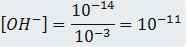5) POH = – log [OH] = 11

pH = 14–11 = 3

pH = 3

Example: How much water must be added to a 10l 0.2 Molar solution weak acid for degree of dissociated to be doubled if Ka = 1.8 × 10–6

HAH+ + A

C – Cα                 Cα   Cα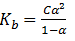After neglection, 1.8 × 10–6 = .2α2

α = .003

Now, Kα = Cα22

Acc. To question, α2 = 2α1

Hence, Ka = C(2α1)2 = C × 4α12

1.8 × 10–6 = C × 4(3)2× 10–6

C = .05

M1V1 = M2V2

10 × 0.2 = .05 V2

V2 = 40l

10l is added initially. Hence we need to add 30l more in order to get the degree of dissociation.

Dissociation of weak base:

Any species which given hydroxyl ion in solution, is called weak base

NH4OHNH4+ + OH

At t = 0           C                        –            –

At t = teq          C – Cα              Cα       Cα

If α<<< 1

Kb =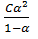Then, Kb = Cα2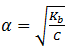[NH4OH] = C – Cα ≈ C

Hence, [NH4+] = Cα =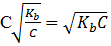[OH] =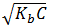POH = – log[OH]

POH =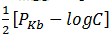Remember, this expression is after consideration α<<< 1.

In other cases, you have to solve the quadratic equation

Example: Calculate the molar concentration of aqueous NH3 solution that has pH = 12 and Kb = 1.8 × 10–5.

Solution:         pH = –log [H+]

12 = – log [H+]

So,                   [H+] = 10–12

NH4OHNH4+ + OH

C(1 - α)        Cα       Cα

K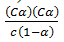K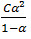Supposse,        α<<< 1

1.8 ×105 = Cα2

α = 1.8 × 103

Now,   [OH] = Cα

[OH][H+] = 1014

[OH] = 102

Cα = 10–2

C =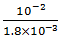= 5.55
C = 5.55

Molar Concentration of NH4OH = C – Cα ≈ C

Molar concentratuion of NH4OH = 5.55mol/l

Ionic Product of Water:

H2OH+ + OH

KW = [H+][OH] = 10–14  at 250C

As dissociation of water is an endothermic reaction. So increase in temperature leads to increase in KW.

At 250C

pH = 7 pOH                Neutral solution

pH< 7 or pOH > 7      Acidic solution

pH> 7 or pOH < 7      Basic solution

Ionic product of water is an equilibrium constant so it depends only on temperature. Hence, ionic product of water is always a constant whatever has been dissolved in water.

At 250C,          Kw = 10–14

(Cα)2 = 10–14

α = 1.8 × 10–9

% dissociation = 1.8 x 107

Effect of temperature on Ionic product of water

As mentioned earlier, with increase in temperature value of equilibrium constant increases.

Temperature                Kw

250C                            10–14

630C                            10–13

900C                            10–12

pH scale and its significance:

• Acidic strength means the tendency of an acid to give H3O+ or H+ in water.

• The concentration of H+ ions is written in a simplified form introduced by Sorenson known as pH scale.

pH = –log H+

• The pH scale was marked from 0 to 14 with central point at 7 at 250 taking water as solvent.

• If the Temperature and solvent are changed, the pH range of the scale will also change.

0-14                 at 250C (Kw = 10–14) Neutral at pH = 7

0-13                 at 800C (Kw = 10–13) Neutral at pH = 6.5

The document Introduction to Ionic Equilibrium Notes | Study Physical Chemistry - Chemistry is a part of the Chemistry Course Physical Chemistry.
All you need of Chemistry at this link: ChemistryUse Code STAYHOME200 and get INR 200 additional OFF Use Coupon CodePhysical Chemistry

84 videos|106 docs|31 tests

Track your progress, build streaks, highlight & save important lessons and more!

,

,

,

,

,

,

,

,

,

,

,

,

,

,

,

,

,

,

,

,

,

;